# Logic Lecture 2 Limitation of Propositional Logic Propositional

• Slides: 28Logic Lecture 2Limitation of Propositional Logic Propositional logic: the world is described in terms of elementary propositions and their logical combinations Elementary statements: • Typically refer to objects, their properties and relations. • But these are not explicitly represented in the propositional logic • Hasan is a NSU student Hasan A NSU student - object - a property Objects and properties are hidden in the statement, it is not possible to reason about themLimitation of Propositional Logic Statements that must be repeated for many objects Example If Hasan is a CSE GUB graduate then Hasan has passed CSE 105 Translation Hasan is a CSE GUB graduate → Hasan has passed CSE 105 Solution: make statements with variables If x is a CSE GUB graduate then x has passed CSE 105 x a CSE GUB graduate → x has passed CSE 105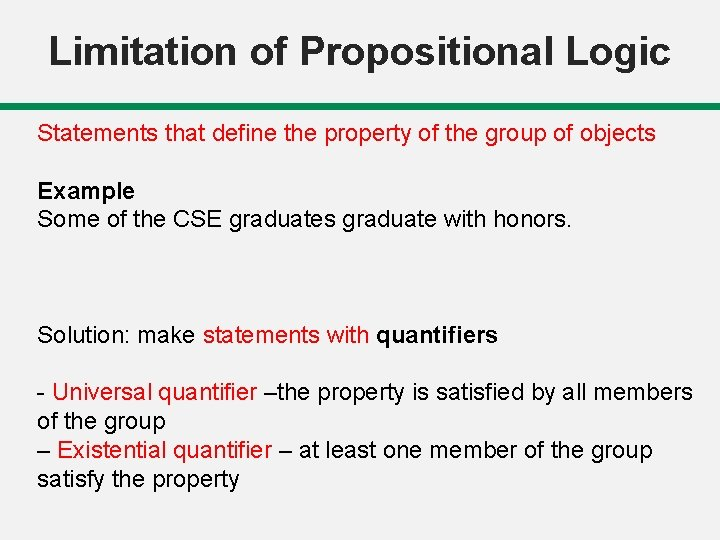Limitation of Propositional Logic Statements that define the property of the group of objects Example Some of the CSE graduates graduate with honors. Solution: make statements with quantifiers - Universal quantifier –the property is satisfied by all members of the group – Existential quantifier – at least one member of the group satisfy the property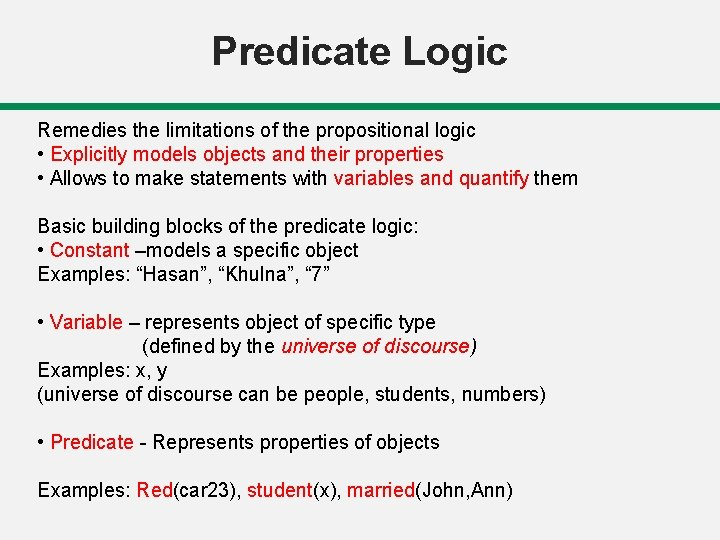Predicate Logic Remedies the limitations of the propositional logic • Explicitly models objects and their properties • Allows to make statements with variables and quantify them Basic building blocks of the predicate logic: • Constant –models a specific object Examples: “Hasan”, “Khulna”, “ 7” • Variable – represents object of specific type (defined by the universe of discourse) Examples: x, y (universe of discourse can be people, students, numbers) • Predicate - Represents properties of objects Examples: Red(car 23), student(x), married(John, Ann)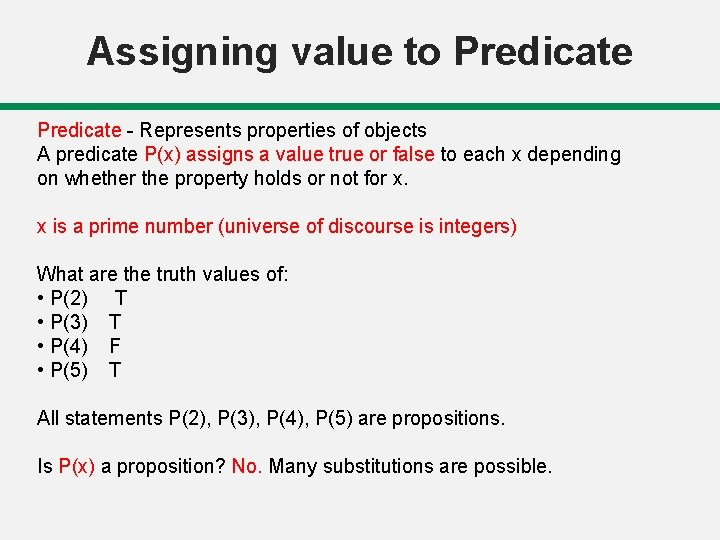Assigning value to Predicate - Represents properties of objects A predicate P(x) assigns a value true or false to each x depending on whether the property holds or not for x. x is a prime number (universe of discourse is integers) What are the truth values of: • P(2) T • P(3) T • P(4) F • P(5) T All statements P(2), P(3), P(4), P(5) are propositions. Is P(x) a proposition? No. Many substitutions are possible.Assigning value to Predicates can have more arguments which represent the relations between objects Example: • Let Q(x, y) denote ‘x+5 >y’ – Is Q(x, y) a proposition? No! – Is Q(3, 7) a proposition? Yes – What is the truth value of: – Q(3, 7) T – Q(1, 6) F - Q(3, y) may be T or F. not a proposition.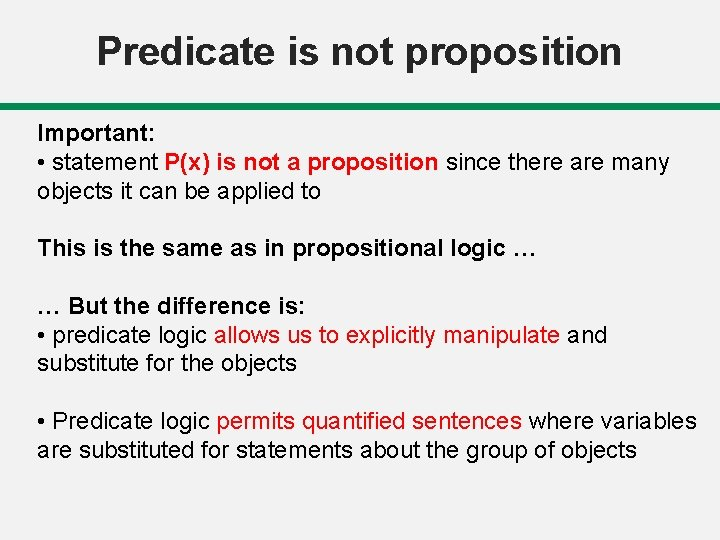Predicate is not proposition Important: • statement P(x) is not a proposition since there are many objects it can be applied to This is the same as in propositional logic … … But the difference is: • predicate logic allows us to explicitly manipulate and substitute for the objects • Predicate logic permits quantified sentences where variables are substituted for statements about the group of objects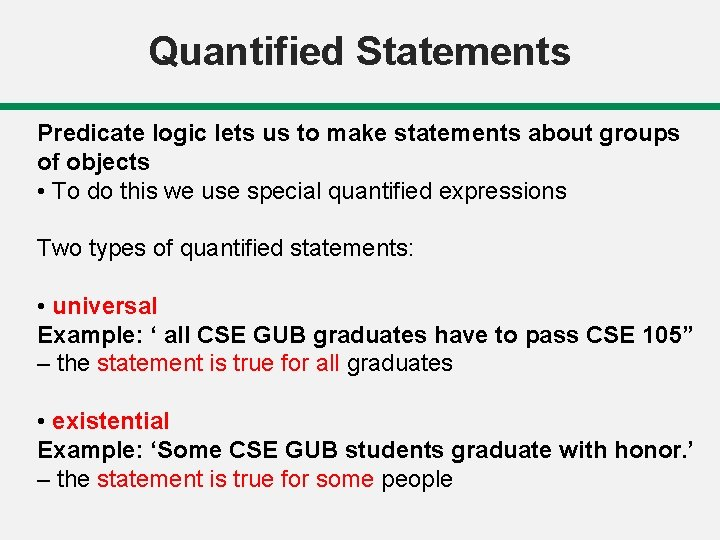Quantified Statements Predicate logic lets us to make statements about groups of objects • To do this we use special quantified expressions Two types of quantified statements: • universal Example: ‘ all CSE GUB graduates have to pass CSE 105” – the statement is true for all graduates • existential Example: ‘Some CSE GUB students graduate with honor. ’ – the statement is true for some peopleUniversal Quantifier Defn: The universal quantification of P(x) is the proposition: "P(x) is true for all values of x in the domain of discourse. " The notation denotes the universal quantification of P(x), and is expressed as for every x, P(x). Example: • Let P(x) denote x > x - 1. • What is the truth value of ? • Assume the universe of discourse of x is all real numbers. • Answer: Since every number x is greater than itself minus 1. Therefore, is true.Universally Quantified Statement Predicate logic lets us make statements about groups of objects Universally quantified statement • CSE-major(x) → Student(x) – Translation: “if x is a CSE-major then x is a student” – Proposition: no. • – Translation: “(For all people it holds that) if a person is a CSE-major then s/he is a student. ” – Proposition: yes.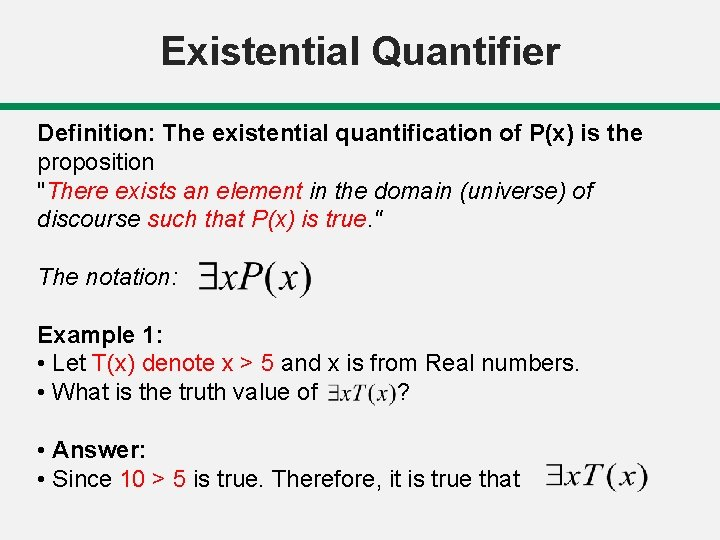Existential Quantifier Definition: The existential quantification of P(x) is the proposition "There exists an element in the domain (universe) of discourse such that P(x) is true. " The notation: Example 1: • Let T(x) denote x > 5 and x is from Real numbers. • What is the truth value of ? • Answer: • Since 10 > 5 is true. Therefore, it is true thatQuantified statements Statements about groups of objects Example: • CSE-NSU-graduate (x) ˄ Honor-student(x) – Translation: “x is a CSE-GUB-graduate and x is an honor student” – Proposition: no. • – Translation: “There is a person who is a CSE-GUBgraduate and who is also an honor student. ” – Proposition: ? yes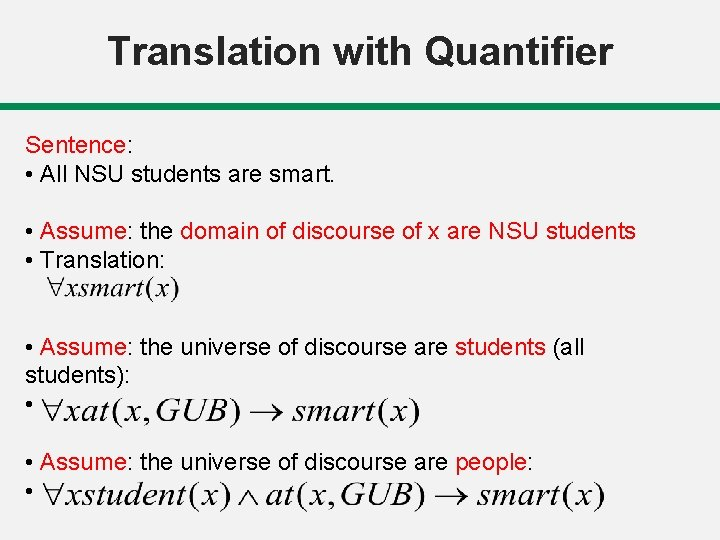Translation with Quantifier Sentence: • All NSU students are smart. • Assume: the domain of discourse of x are NSU students • Translation: • Assume: the universe of discourse are students (all students): • • Assume: the universe of discourse are people: •Translation with Quantifier Sentence: • Someone at GUB is smart. • Assume: the domain of discourse are all GUB affiliates • Translation: • Assume: the universe of discourse are people: •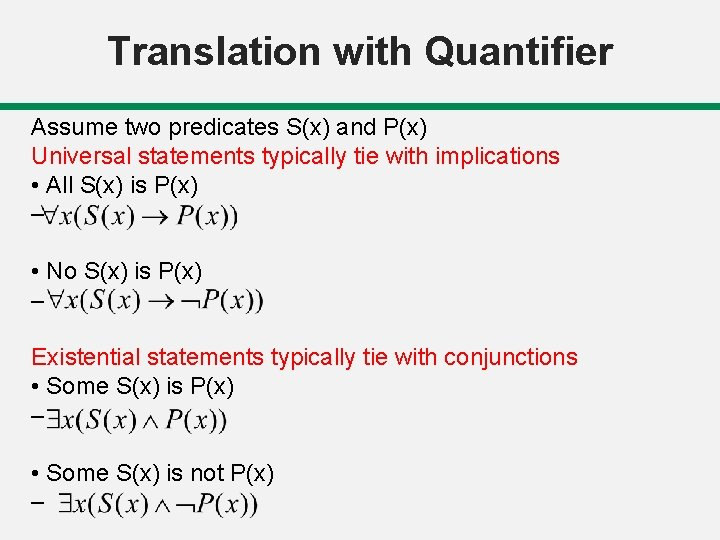Translation with Quantifier Assume two predicates S(x) and P(x) Universal statements typically tie with implications • All S(x) is P(x) – • No S(x) is P(x) – Existential statements typically tie with conjunctions • Some S(x) is P(x) – • Some S(x) is not P(x) –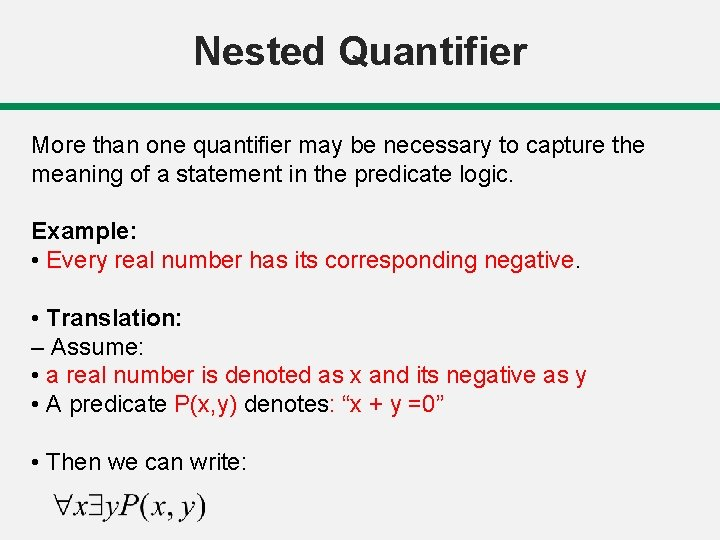Nested Quantifier More than one quantifier may be necessary to capture the meaning of a statement in the predicate logic. Example: • Every real number has its corresponding negative. • Translation: – Assume: • a real number is denoted as x and its negative as y • A predicate P(x, y) denotes: “x + y =0” • Then we can write: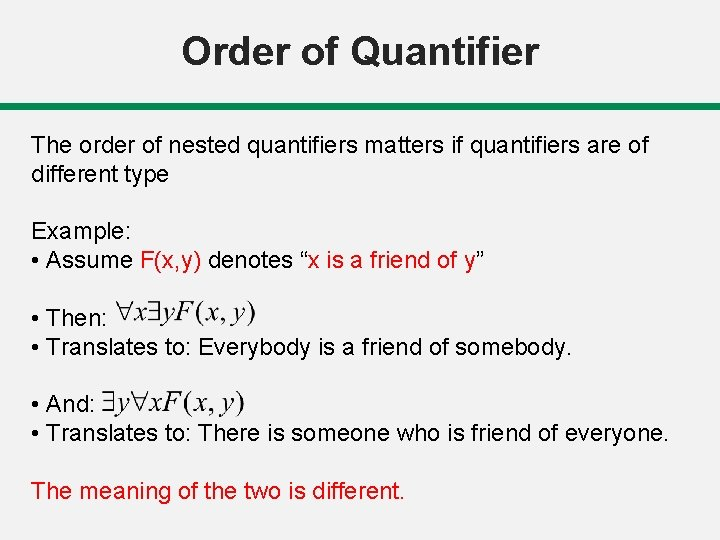Order of Quantifier The order of nested quantifiers matters if quantifiers are of different type Example: • Assume F(x, y) denotes “x is a friend of y” • Then: • Translates to: Everybody is a friend of somebody. • And: • Translates to: There is someone who is friend of everyone. The meaning of the two is different.Order of Quantifier The order of nested quantifiers does not matter if quantifiers are of the same type Example: • For all x and y, if x is a parent of y then y is a child of x • Assume: – Parent(x, y) denotes “x is a parent of y” – Child(x, y) denotes “x is a child of y” • Two equivalent ways to represent the statement:Negation of quantifiers English statement: • Nothing is perfect. • Translation: Another way to express the same meaning: • Everything is imperfect. • Translation: Conclusion: is equivalent to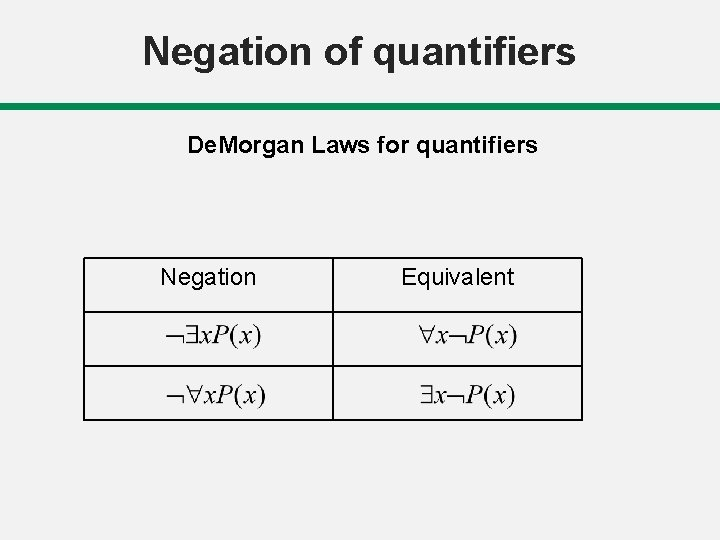Negation of quantifiers De. Morgan Laws for quantifiers Negation Equivalent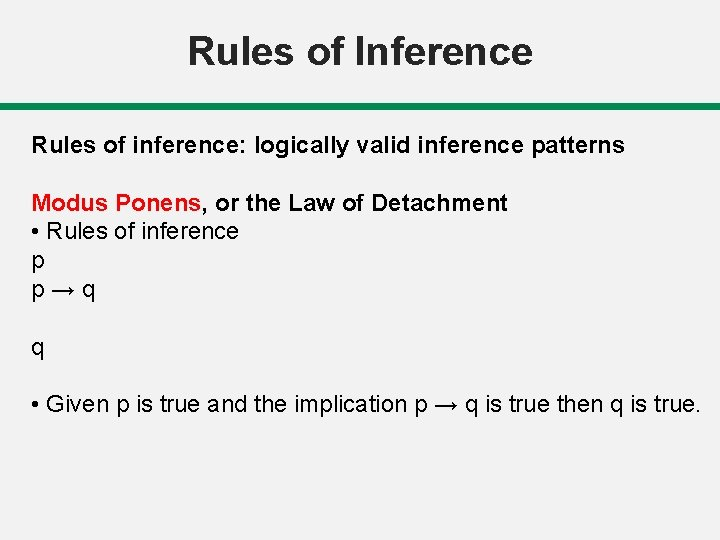Rules of Inference Rules of inference: logically valid inference patterns Modus Ponens, or the Law of Detachment • Rules of inference p p→q q • Given p is true and the implication p → q is true then q is true.Rules of Inference Addition p → (p˅q) p p˅q Simplification p˄q →p p˄q pRules of Inference Modus Tollens [¬q ˄ (p → q)] → ¬p Hypothetical Syllogism [(p → q) ˄ (q → r)] → (p → r) Disjunctive Syllogism [(p ˅ q) ˄ ¬p] → q Logical Equivalence p <=> q p→q is a tautology De. Morgans law ¬( p ˅ q ) <=> ¬p ˄ ¬q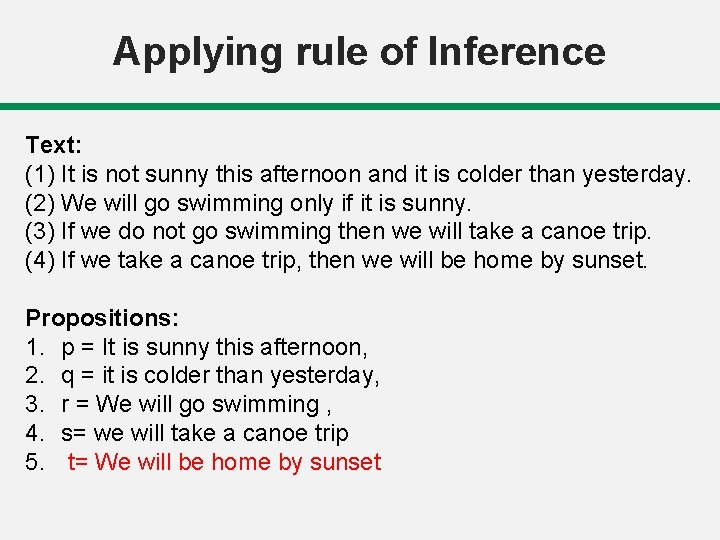Applying rule of Inference Text: (1) It is not sunny this afternoon and it is colder than yesterday. (2) We will go swimming only if it is sunny. (3) If we do not go swimming then we will take a canoe trip. (4) If we take a canoe trip, then we will be home by sunset. Propositions: 1. p = It is sunny this afternoon, 2. q = it is colder than yesterday, 3. r = We will go swimming , 4. s= we will take a canoe trip 5. t= We will be home by sunset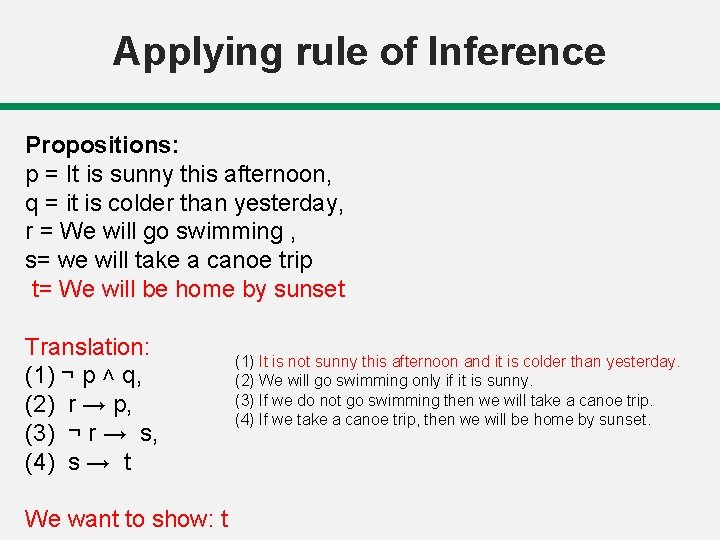Applying rule of Inference Propositions: p = It is sunny this afternoon, q = it is colder than yesterday, r = We will go swimming , s= we will take a canoe trip t= We will be home by sunset Translation: (1) ¬ p ˄ q, (2) r → p, (3) ¬ r → s, (4) s → t We want to show: t (1) It is not sunny this afternoon and it is colder than yesterday. (2) We will go swimming only if it is sunny. (3) If we do not go swimming then we will take a canoe trip. (4) If we take a canoe trip, then we will be home by sunset.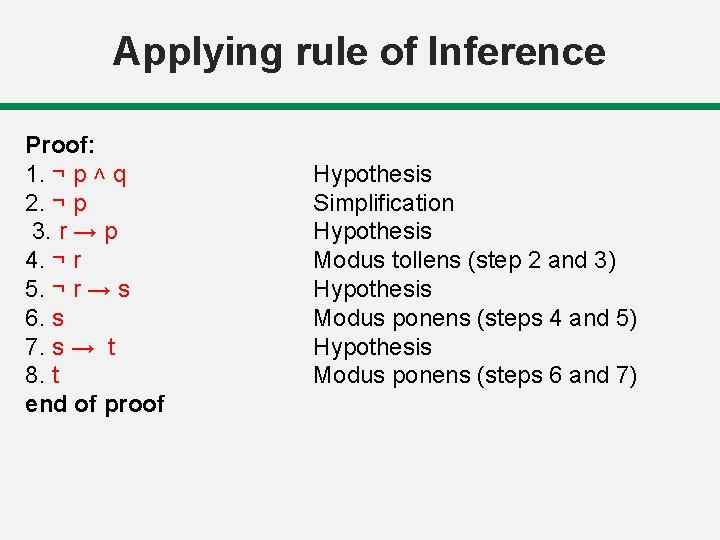Applying rule of Inference Proof: 1. ¬ p ˄ q 2. ¬ p 3. r → p 4. ¬ r 5. ¬ r → s 6. s 7. s → t 8. t end of proof Hypothesis Simplification Hypothesis Modus tollens (step 2 and 3) Hypothesis Modus ponens (steps 4 and 5) Hypothesis Modus ponens (steps 6 and 7)Thank You